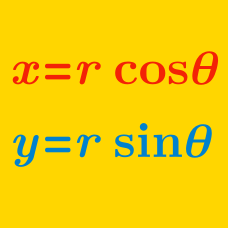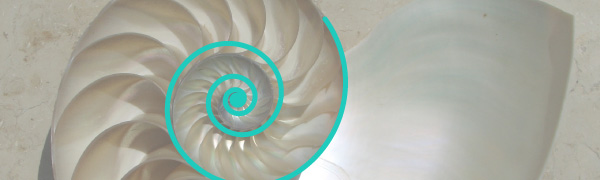Algebra

# Polar Coordinates: Level 3 Challenges

If the center of a regular hexagon is at the origin and one of the vertices on the Argand plane is $1 + 2i$, then what is its perimeter?The Nautilus Spiral is given by the polar coordinates $r = e ^ \theta$. Its shape resembles that of the Nautilus Shell, hence its name.

$\large \ln\left(\dfrac{\omega^\omega}{\omega^{\omega^2}}\right)$

If $\omega$ is a non-real complex cube root of unity, then what is the value of the expression above?

$\large \ln {( i) } = \ ?$

What do we obtain when we multiply the polar coordinates:

$( r_1 , \theta _1 ) \times ( r_2 , \theta _2 ) ?$

×